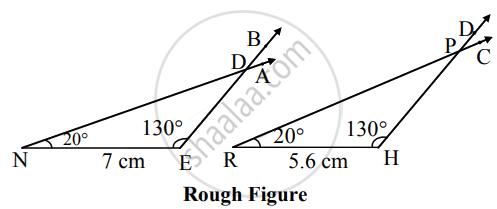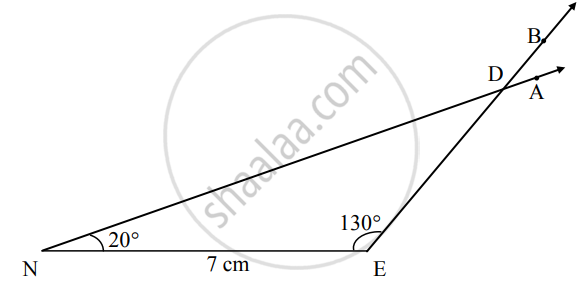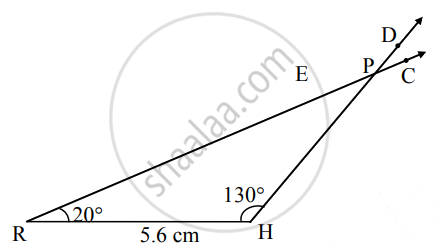# ΔRHP ~ ΔNED, In ΔNED, NE = 7 cm. ∠D = 30°, ∠N = 20°, HPEDHPED=45, then construct ΔRHP and ∆NED - Geometry

Diagram

ΔRHP ~ ΔNED, In ΔNED, NE = 7 cm. ∠D = 30°, ∠N = 20°, "HP"/"ED" = 4/5, then construct ΔRHP and ∆NED

#### SolutionAnalysis:

In ∆NED, ∠D = 30° and ∠N = 20°   ......[Given]

∴ ∠E = 130°    ......(ii) [Remaining angle of a triangle]

∆RHP ∼ ∆NED

∴ "RH"/"NE" = "HP"/"ED" = "PR"/"DN"  ......[Corresponding sides of similar triangles]

∴ "RH"/7 = 4/5    ......[Given]

∴ RH = (4 xx 7)/5 = 5.6 cm

Also, ∠R = ∠N, ∠H = ∠E, ∠P = ∠D   ......(iiii) [Corresponding angles of similar triangles]

∴ ∠R = 20°, ∠H = 130°, ∠P = 30°    ......[From (i), (ii) and (iii)]Steps of construction:

 ∆NED ∆RHP i. Draw seg NE of 7 cm Draw seg RH of 5.6 cm ii. Draw a ray NA and EB such that ∠ANE = 20° and ∠BEN = 130°. Draw a ray RC and HD such that ∠CRH = 20° and ∠DHR = 130°. iii. Name the point of intersection of rays D. Name the point of intersection of rays P.
Concept: Division of a Line Segment
Is there an error in this question or solution?

Share